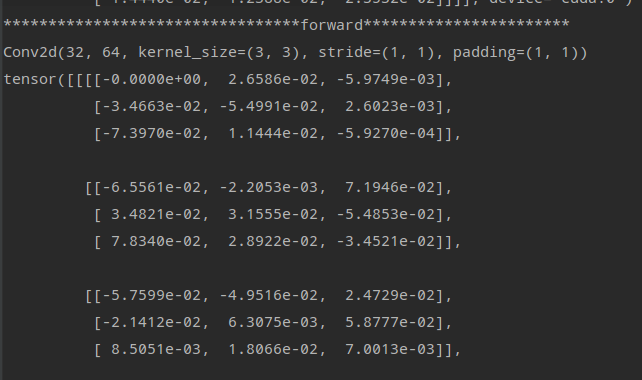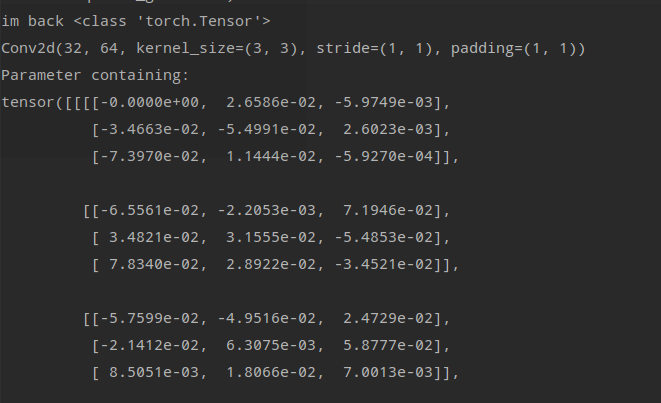# Update some weight with torch.no_grad and type(weight.grad) is Nonetype

Hi all,

I’m trying to freeze some weights(value = 0) from updating during backward, but I failed for several timesThe method one I tried is using trying to zero out the gradient with below codes:

``````    def hook(self, model, inputs):
print('*********************************forward***********************')
print(model)
print(model.weight.data )
model.weight.data = model.weight.data * self.sparse_mask[self.type[model]]

print(model)
print(model.weight)

def register_hook(self,module):
self.handle = module.register_forward_pre_hook(self.hook)
self.backhandle = module.register_backward_hook(self.back_hook)

for m in model.modules():
if isinstance(m, nn.Conv2d):
sp.register_hook(m)

pred = model(data)
loss = criterion(pred, target)
losses.update(loss.data.cpu().numpy())
loss.backward()
``````

But I got this error

TypeError: mul(): argument ‘other’ (position 1) must be Tensor, not NoneType

And my output of `back_hook()` is

im back NoneType which means my weight.grad is None

I don’t have any clue why this happened!

The method 2 I tried is

``````    def back_hook(self, model,grad_input,grad_output):
model.weight.data = model.weight.data * self.sparse_mask[self.type[model]]

``````

And keeps others as the same.

There is no bug and error this time, however, the output is not correct.
This is the backward of iter2These two are the forward of iter3This is the backward of iter3I can’t figure out Why in the first pic of forward part the others weight are not updating except the left-top one, from 0 to -1.1226e-3?

But what I want is freeze that weight which value = 0 and update the others non-zero weight.

Anyone would like to help me solve that problem?

Really appreciate!